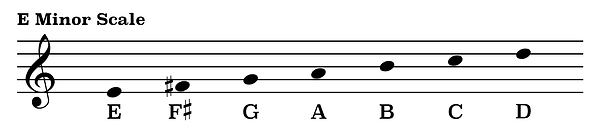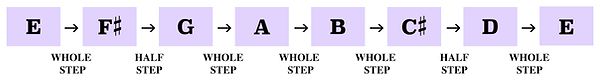top of page

## Mode Construction | Dorian Mode (2 of 7)

The notes of Dorian mode are essentially the same as the notes of a natural minor scale with the exception of a raised sixth scale degree. For this reason, Dorian mode can be used in both Major and minor settings.

Here are the notes of an A minor scale.If we raise the sixth scale degree, F, one half step to the note F-sharp, we will have changed the scale from A minor to A Dorian.Likewise, the sixth scale degree of an E minor scale is the note C natural.If we raised that note one half step to the note C-sharp we will have created an E Dorian scale.As we learned in the previous video, the notes of a Dorian mode can be derived from the second scale degree of a corresponding Major scale.

For example, a C Major scale played from the second scale degree, D, to the next note D one octave above is called D Dorian.In that same respect, the second scale degree of a G Major scale is the note A. Therefore, the notes of the A Dorian scale can be played in the key of G Major from the note A to the next A one octave above.This same method of finding and playing the Dorian mode may be applied to any Major scale.

Another way to think about Dorian mode is by its unique pattern of whole steps and half steps.

First, here is the pattern of steps which produces a Major scale.Knowing that the Dorian mode starts on the second scale degree of a Major scale the order of steps which produces the Dorian mode is then whole step, half step, whole step, whole step, whole step, half step, whole step.The Dorian mode is unique in the fact that it is the only mode whose pattern of steps is the same played ascending or descending.

Let's use this pattern of steps to construct the notes of an E Dorian scale.A whole step above the note E is the note F-sharp. A half step above F-sharp is the note G. G to the note A is a whole step. A whole step above A is the note B. B to C-sharp is another whole step. A half step above the note C-sharp is the note D. And finally a whole step above D is the note E.

Again, this pattern of steps may be used to construct a Dorian mode above any given note.

bottom of page